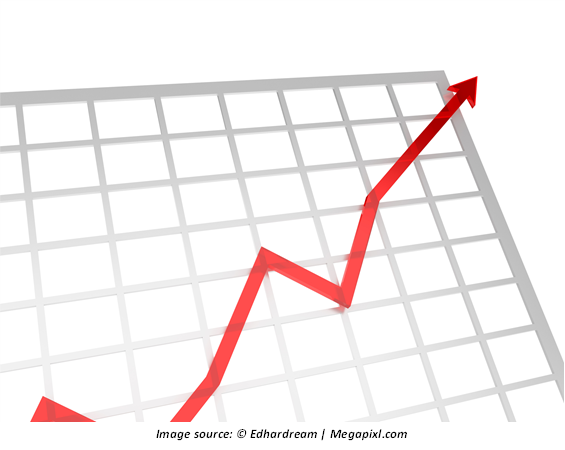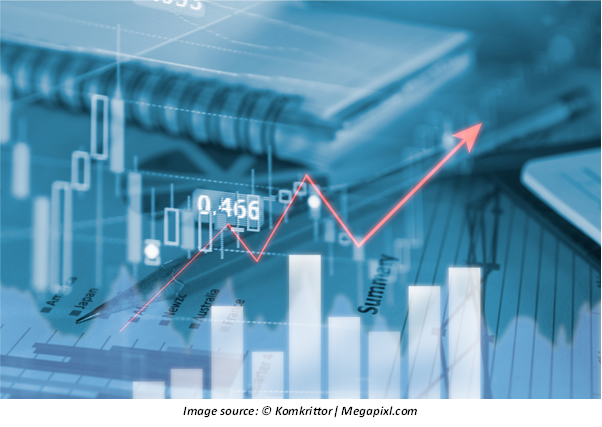# Risk Curve

• Updated on

## What is the risk curve?

The graphical presentation of the variables risk and returns is known as the risk curve. Chiefly, two variables are plotted on the graph which represents the risk and returns associated with a financial instrument.The risk curve creates a visual presentation of the returns which can be enjoyed by the investor for undertaking a specific level of risk. The risk type can be indicated in the graph or risk curve. Generally, a risk curve is made up of multiple data points that depict the multiple assets, it aids the visual representation in terms of the returns and risks associated with different financial assets. The risk curve is a crucial metric in the Capital Asset Pricing Model (CAPM) and mean variance analysis.

Summary
• The graphical presentation of variables risk and returns is known as the risk curve.
• The variables are plotted on the graph which represents the risk and returns associated with a financial instrument.
• The risk curve creates a visual presentation of the returns which can be enjoyed by the investor for undertaking a specific level of risk.
• The risk curve is a crucial metric in the Capital Asset Pricing Model (CAPM) and mean variance analysis.

## How does the risk curve work?

The risk curve is employed to present the returns and risks of dissimilar financial assets or similar classes of financial assets. Mostly, the x-axis of the graph presents the risk, and the y-axis presents the expected or average return from the investment. Generally, the risk curve is in balloon shape when the financial assets have both higher risk and higher expected returns from investment. The graph contracts when the risk and return are lower from a specific investment.

What is risk curve?

To illustrate, a risk-free financial investment asset such as the Treasury bills or government bonds will be located at the lower-left corner of the graph. Whereas high risk assets such as small-cap stock or leveraged ETF will be located at the top-right of the graph.

The assets that have a higher risk associated with them are generally expected to give a higher rate of return. This signifies that there exists a trade-off between the expected returns and the level of risk.

## What is the application of the risk curve?

Two variables are generally plotted on the graph, the x-axis presents the risks (vertical axis), and the y-axis (horizontal axis) highlights the expected rate of return on the investment. The assets which are presented on the graphs, through the risk curve, can be both similar assets and dissimilar assets. For the assets that have a low level of risk or are risk-free with a lower level of return, the curve is located at the lower end at the left side of the graph. On other hand, assets with a high level of risk and return profile are located at the top right corner. Generally, ETFs are located at the top right corner of the graph.## How is risk curve used in efficient frontier and MPT?

Risk curve is used in the Modern portfolio theory to indicate the benefits associated with a range of portfolios across the efficient frontier. Portfolios which are located below the efficient frontier or the curve are sub-optimal. These portfolios do not offer expected returns which are enough as per the specific level of risk.

A portfolio that is positioned at the right lower corner is considered sub-optimal because the return provided from these portfolios is less than the return provided by the other portfolios for the same level of risk.

It should be noted that risk and return calculations are based on historical data.

## What are the special points which should be considered while employing a risk curve?

The data which is used for the creation of the risk curve is historical data for different financial assets or historical standard deviations.

The risk curve on the graph presents the risk and the return which were delivered by the financial asset in the past. The historical time has an impact on the curve. Moreover, the curve is not efficient in making any future predictions, as the risk and return profile changes on a regular and daily basis.

## What is yield curve risk?

The probability of a shift in the yield curve and the shift that affects the securities’ value which is tied with interest rate is known as the yield curve risk.

## What is the working of yield curve risk?

Yield curve risk is also referred to as the term structure of interest rate. In the yield curve, the yield of similar types of bonds is plotted against their maturities. The graphs which include the variables – coupon rate and maturities are known as spot curves.

A positive yield curve is a line that goes up to the right, that is, investors require a higher rate of interest for investing for longer durations. An inverted yield curve is a downward line and yield curve risk can also be flat. The shape of the curve helps in comparing the yields of the short term and long term bonds. The curve also projects the economic growth and future interest rates.

Thus, the yield curve can be utilised by the investors while constructing their portfolios. They will be able to make predictions regarding the rate of returns and determine the way their portfolio will react with the change in the interest rates.

An investor is exposed to the yield curve risk if they hold nay security which is backed by interest rate. Banks are immensely affected by the yield curve risk. However, it is difficult to predict the movement of the yield curve. Generally, it is assumed that all the curves move in a similar way, that is, the shape remains same, and curve only moves down or up.

##### Top ASX Listed Companies

Definition:
We use cookies to ensure that we give you the best experience on our website. If you continue to use this site we will assume that you are happy with it. OK